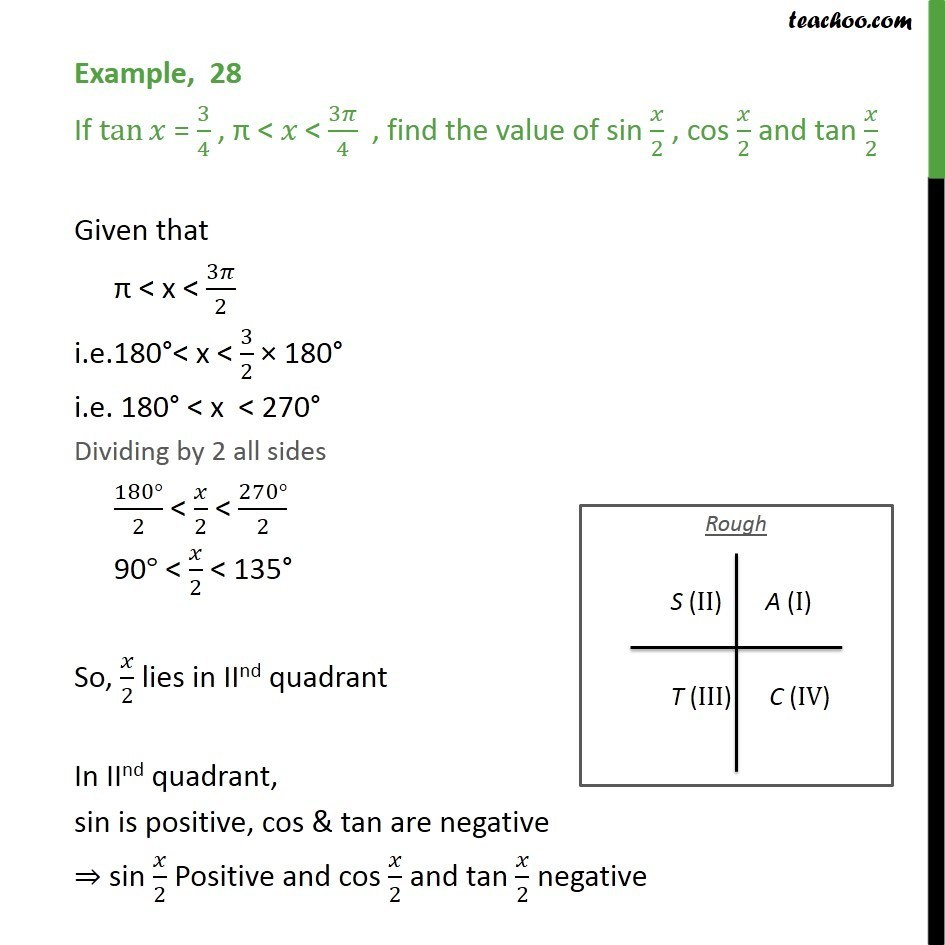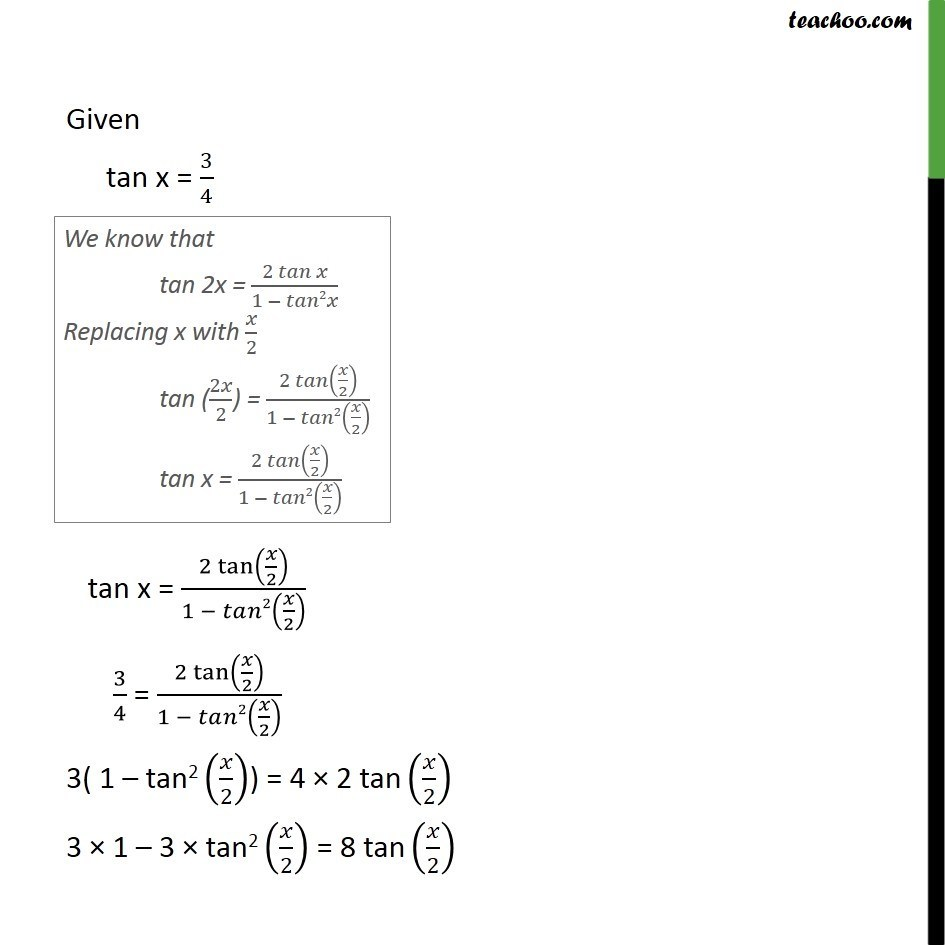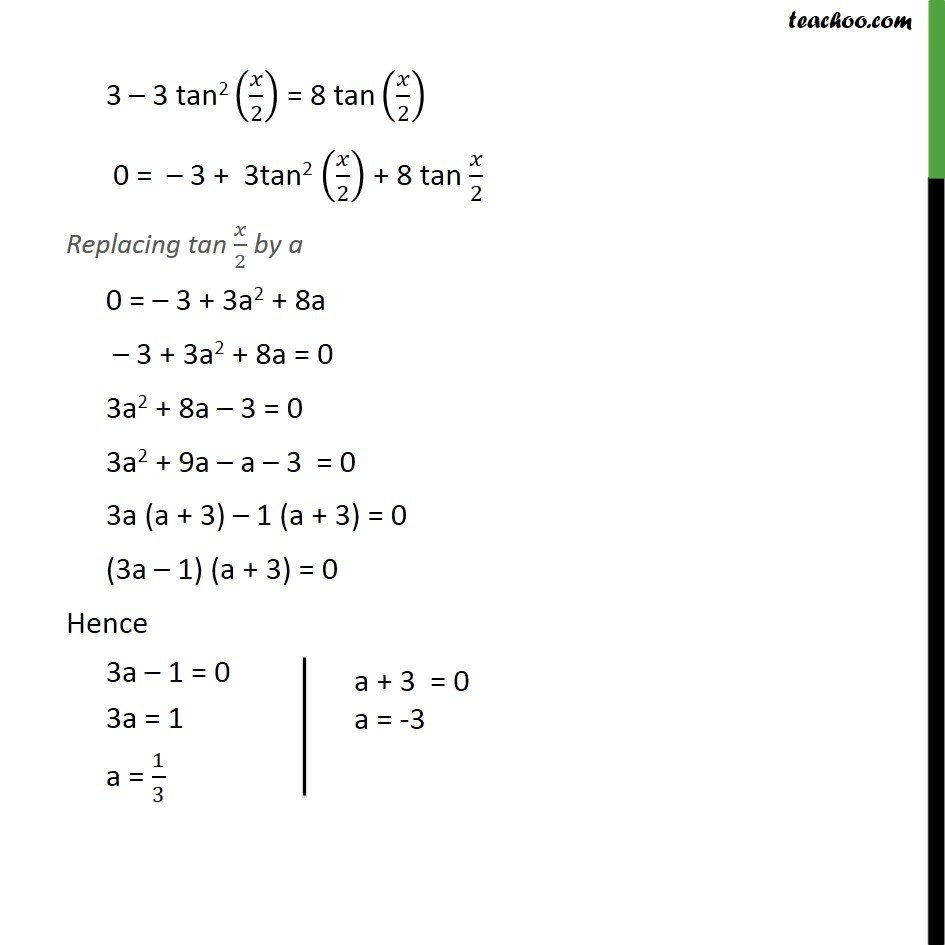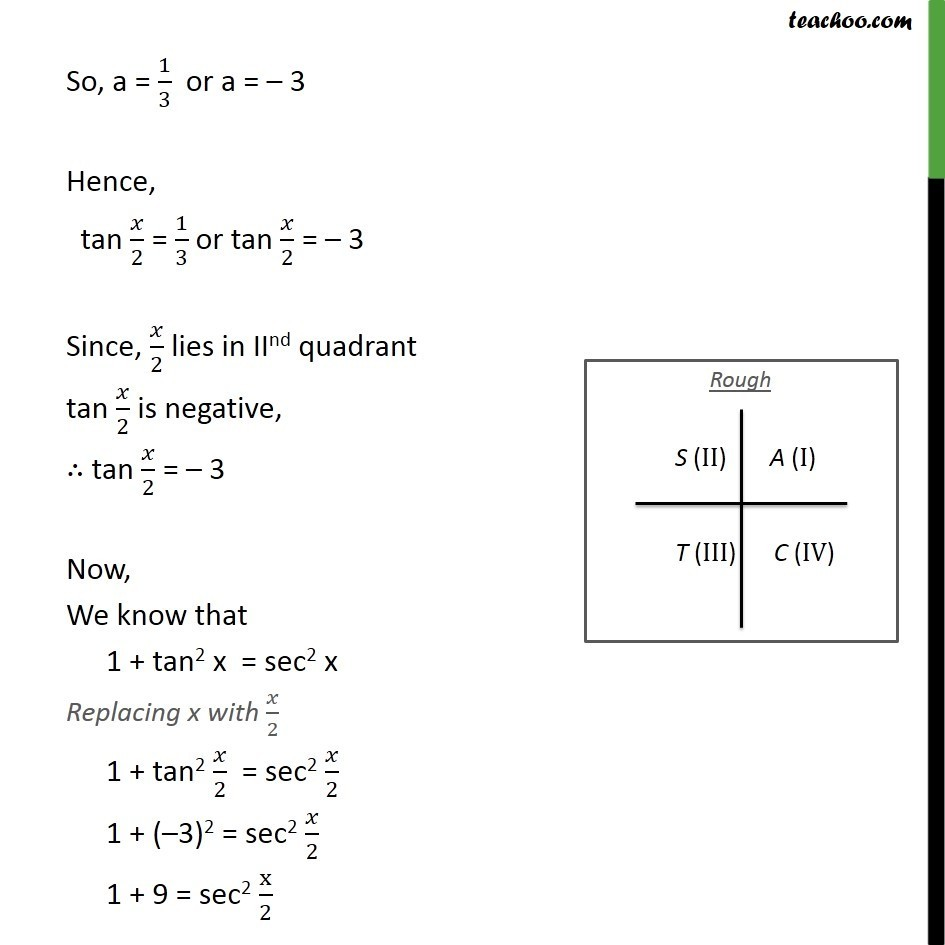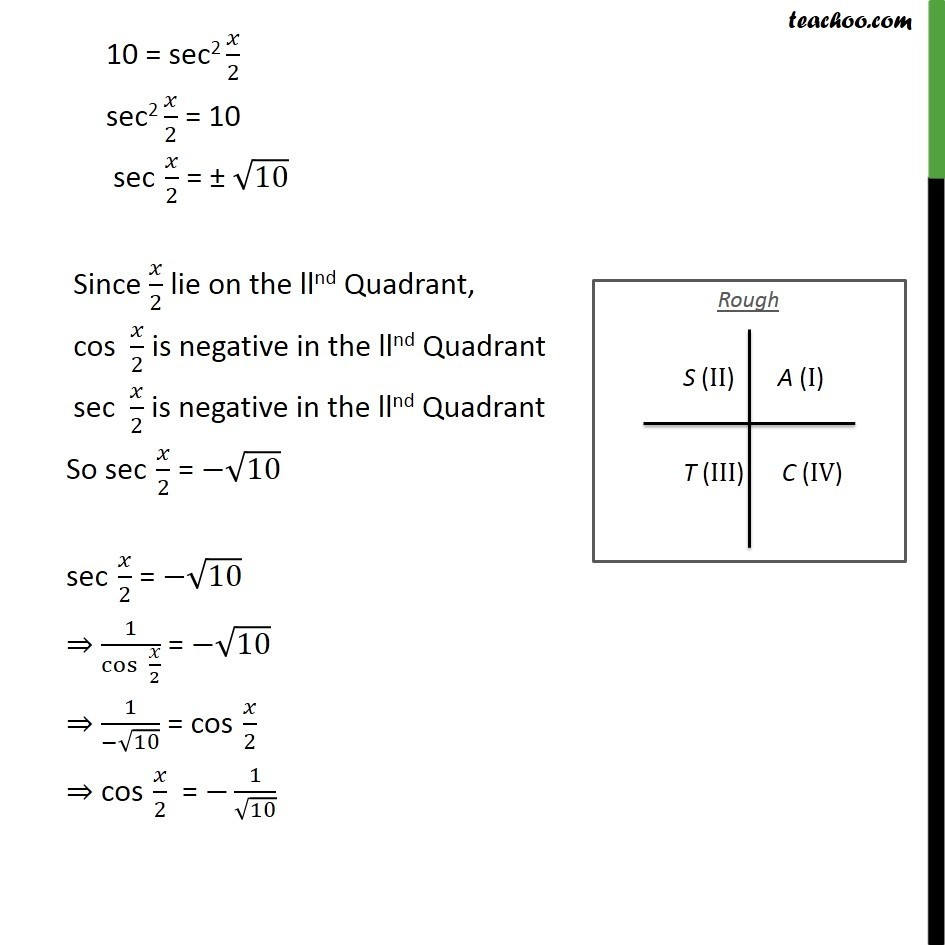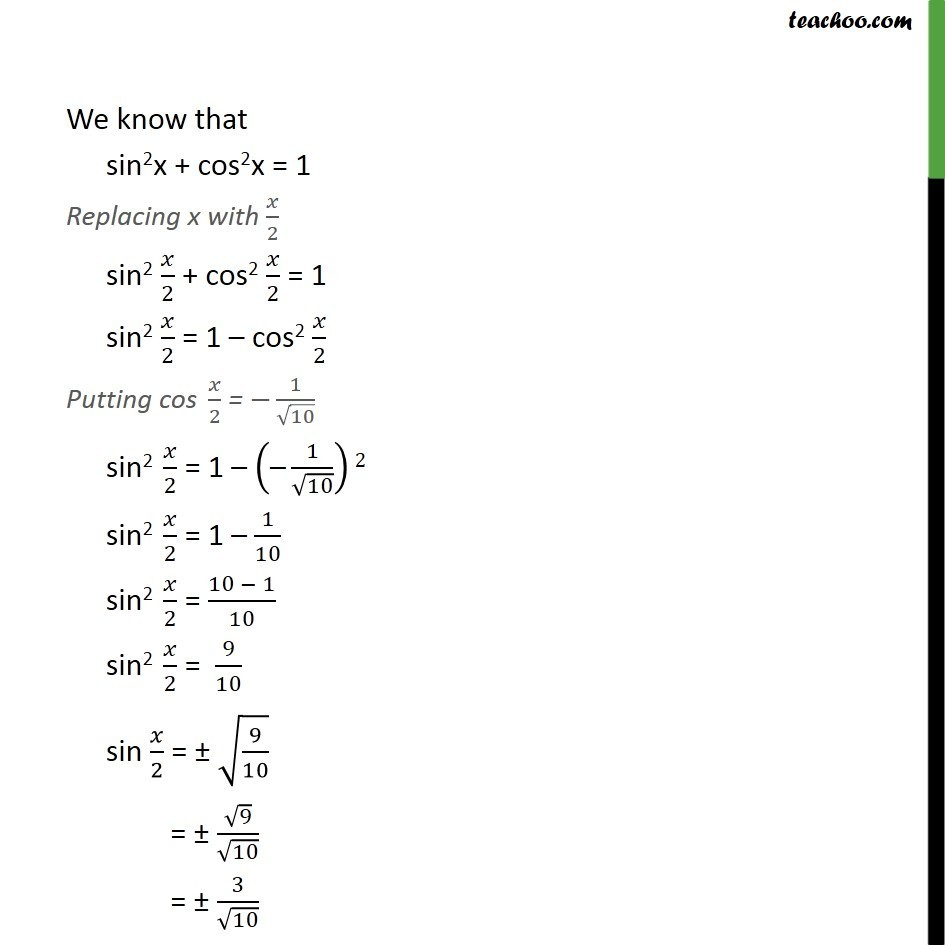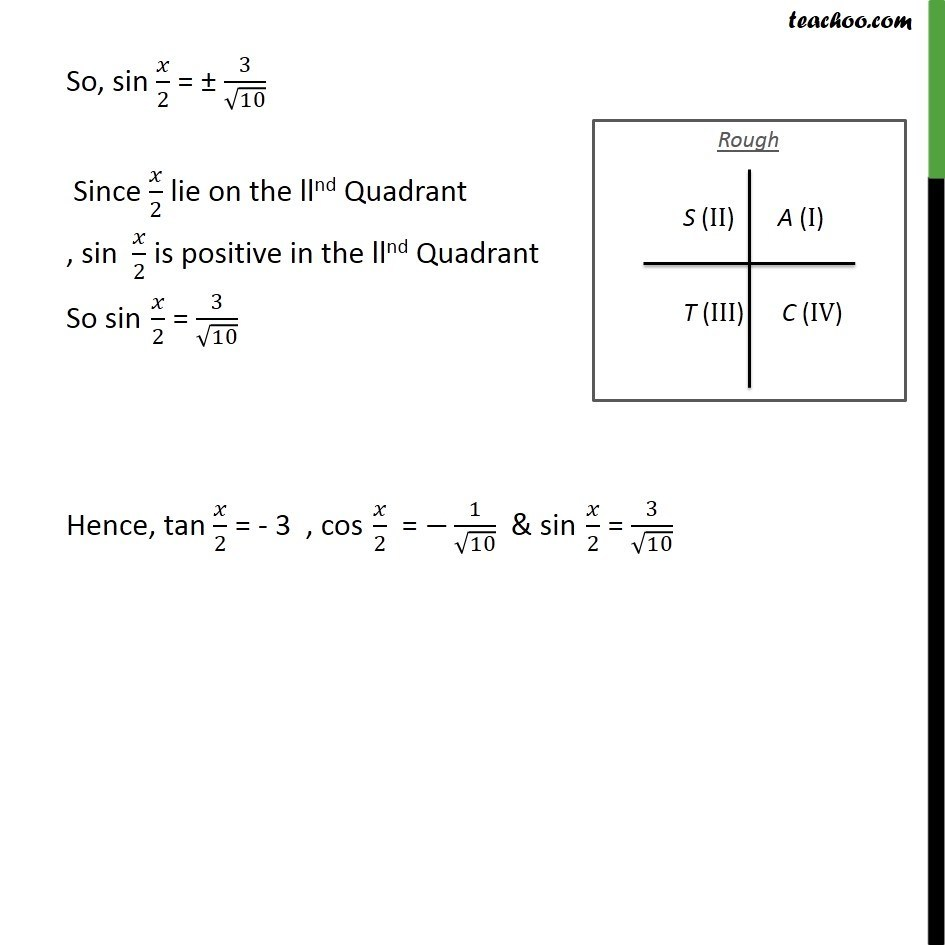1. Chapter 3 Class 11 Trigonometric Functions
2. Concept wise
3. 2x 3x formula - Finding value

Transcript

Example, 28 If tan⁡𝑥 = 3/4 , "π" < 𝑥 < 3𝜋/4 , find the value of sin 𝑥/2 , cos 𝑥/2 and tan 𝑥/2 Given that "π" < x < 3𝜋/2 i.e.180°< x < 3/2 × 180° i.e. 180° < x < 270° Dividing by 2 all sides (180°)/2 < 𝑥/2 < (270°)/2 90° < 𝑥/2 < 135° So, 𝑥/2 lies in IInd quadrant In IInd quadrant, sin is positive, cos & tan are negative ⇒ sin 𝑥/2 Positive and cos 𝑥/2 and tan 𝑥/2 negative Given tan x = 3/4 tan x = (2 tan⁡(𝑥/2))/(1 − 𝑡𝑎𝑛2(𝑥/2) ) 3/4 = (2 tan⁡(𝑥/2))/(1 − 𝑡𝑎𝑛2(𝑥/2) ) 3( 1 – tan2 (𝑥/2)) = 4 × 2 tan (𝑥/2) 3 × 1 – 3 × tan2 (𝑥/2) = 8 tan (𝑥/2) 3 – 3 tan2 (𝑥/2) = 8 tan (𝑥/2) 0 = – 3 + 3tan2 (𝑥/2) + 8 tan 𝑥/2 Replacing tan 𝑥/2 by a 0 = – 3 + 3a2 + 8a – 3 + 3a2 + 8a = 0 3a2 + 8a – 3 = 0 3a2 + 9a – a – 3 = 0 3a (a + 3) – 1 (a + 3) = 0 (3a – 1) (a + 3) = 0 Hence 3a – 1 = 0 3a = 1 a = 1/3 So, a = 1/3 or a = – 3 Hence, tan 𝑥/2 = 1/3 or tan 𝑥/2 = – 3 Since, 𝑥/2 lies in IInd quadrant tan 𝑥/2 is negative, ∴ tan 𝑥/2 = – 3 Now, We know that 1 + tan2 x = sec2 x Replacing x with 𝑥/2 1 + tan2 𝑥/2 = sec2 𝑥/2 1 + (–3)2 = sec2 𝑥/2 1 + 9 = sec2 x/2 10 = sec2 𝑥/2 sec2 𝑥/2 = 10 sec 𝑥/2 = ± √10 Since 𝑥/2 lie on the llnd Quadrant, cos 𝑥/2 is negative in the llnd Quadrant sec 𝑥/2 is negative in the llnd Quadrant So sec 𝑥/2 = −√10 sec 𝑥/2 = −√10 ⇒ 1/cos⁡〖 𝑥/2〗 = −√10 ⇒ 1/(−√10) = cos 𝑥/2 ⇒ cos 𝑥/2 = −1/√10 We know that sin2x + cos2x = 1 Replacing x with 𝑥/2 sin2 𝑥/2 + cos2 𝑥/2 = 1 sin2 𝑥/2 = 1 – cos2 𝑥/2 Putting cos 𝑥/2 = −1/√10 sin2 𝑥/2 = 1 – ("–" 1/√10)2 sin2 𝑥/2 = 1 – 1/10 sin2 𝑥/2 = (10 − 1)/10 sin2 𝑥/2 = 9/10 sin 𝑥/2 = ± √(9/10) = ± √9/√10 = ± 3/√10 So, sin 𝑥/2 = ± 3/√10 Since 𝑥/2 lie on the llnd Quadrant , sin 𝑥/2 is positive in the llnd Quadrant So sin 𝑥/2 = 3/√10 Hence, tan 𝑥/2 = - 3 , cos 𝑥/2 = −1/√10 & sin 𝑥/2 = 3/√10

2x 3x formula - Finding value